# Deep-dive in Bayesian Hyper-Parameter Tuning

An intuition and implementation summary of Sequential Model-Based Optimization Algorithm for Bayesian Hyper-Parameter Tuning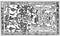Maya Ancient Astronaut

# Complete Feature Selection Techniques 4 - 2 Correlation Analysis

Summarize math intuition and demonstrate Correlation, Multicollinearity and Exploratory Factor Analysis for feature selection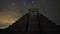Aztec civilization from wallpaperflare

Complete Feature Selection Techniques

1. Statistical Test & Analysis
2. Correlation Analysis
3. Dimension Reduction
4. Model Driven

# Complete Feature Selection Techniques 4 - 3 Dimension Reduction

Summarize math intuition and demonstrate PCA, LDA, MDS, ISOMAP, T-SNE, UMAP for feature dimension reduction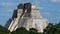Maya Civilization from wallpaperflare

Complete Feature Selection Techniques

1. Statistical Test & Analysis
2. Correlation Analysis
3. Dimension Reduction
4. Model Driven

# PCA and LDA

Both Principal Component Analysis(PCA) and Linear Discriminant Analysis(LDA) are linear transformation techniques.

Linear transformation can scale and rotate data points from original axis(a.k.a space) into a new axis, and the data distribution will be changed accordingly during the scaling and rotating.

So what PCA and LDA do, is under a certain criteria to find the best transformation(scale and rotate).

## Linear Transformation and Orthogonal Projection

The following is an example of Linear Transformation and Orthogonal Projection.

x

# Complete Feature Selection Techniques 4-1 Statistical Test & Analysis

Explain and demonstrate Mutual Information, Chi-Square Test, ANOVA F-Test, Regression t-Test and Variance Check for model feature selection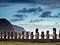Moai statues, Easter Island, Chile

Complete Feature Selection Techniques

1. Statistical Test & Analysis
2. Correlation Analysis
3. Dimension Reduction
4. Model Driven

# Mutual Information (MI)

In statistics, Mutual Information (MI) of two random variables is a measure of the mutual dependence between the two variables. MI is equal to zero if two random variables are independent, and higher values mean higher dependency.

For feature selection, we can use MI to measure the dependency of a feature variable and target variable. MI can be represented as below:

I(x , y) = H(y) - H(y|x)

# Run through LightGBM Fast Training Techniques

A complete explanation for LightGBM - The Fastest Gradient Boosting Model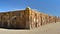Puma Punku, Bolivia

LightGBM is a Gradient Boosting Decision Tree Model(GBDT) developed by Microsoft in 2016, compared with other GBDT models, LightGBM is most featured by its faster training efficiency and great accuracy.

There is no fundamental structure difference between LightGBM and general Gradient Boosting Decision Tree model, but with the following special techniques, LightGBM make itself faster in training.

1. Gradient-based One-Side Sampling(GOSS)
2. Histogram Based Best Value Search in Tree Node Splitting
3. Optimal Split for Categorical Features
4. Exclusive Feature Bundling
5. Leaf-wise Tree Growth Strategy
6. Parallel optimization

# 1. Gradient-based One-Side Sampling(GOSS)

The classic tree based gradient boosting(GBDT)…

# XGBoost Math Intuition Summary

A complete explanation for XGBoost - Most popular Gradient Boosting Model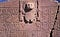Puma Punku, Bolivia

XGBoost is derived from Gradient Boosting Model(GBM), compared with GBM, XGBoost introduces a different way to train the ensemble weaker leaner, so let’s start from here.

Suppose we have the below K trees boosting mode

# Math Intuition Summary on Variational Autoencoder

Detailed explanation on the algorithm of Variational Autoencoder Model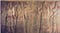Sumerian, The earliest known civilization

My math intuition summary for the Variational Autoencoders (VAEs) will base on the below classical Variational Autoencoders (VAEs) architecture.

# Understand CatBoost Intuition and Training Process

A detailed explanation for CatBoost - Most Delicate Gradient Boosting Model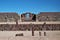Puma Punku, Bolivia

CatBoost is one Gradient Boosting Decision Tree model introduced by Yandex in 2017. Compared with XGBoost and LighGBM, CatBoost is believe to be better in accuracy and easier to use for categorical data.

The technical innovations of CatBoost are

1. Ordered Target Statistics (TS) Encoding for Categorical Feature
2. Ordered Boosting
3. Uses oblivious decision trees and Combining features for tree node splitting

Let’s firstly go through General CatBoost training steps.

# Methods you need know to Estimate Feature Importance for ML models

Introduce and demo how to estimate model feature importance use feature permutation, column drop, SHAP values and model specific metrics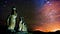Moai statues, Easter Island, Chile from wallpaperflare

One most asked question when review a machine learning mode with business is “what are the major factors impact model prediction?

To response this question, Data scientist always use Feature Importance as part of the answer. So, in this story we will explore Feature Importance and go through three ways to estimate it.

1. Built-in Model Specific Feature Importance
2. Permutation and Drop Importance
3. SHAP values based Feature Importance

One important point regarding the Feature Importance, normally, when we talking about feature…

# Immediately Understand LIME for ML Model Explanation Part 2. LIME for Image and Text Model Interpretation

## Building up intuition for using LIME to interpret Image and Text models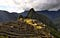Machu Picchu (Lost City of the Incas), Peru from https://www.ivsky.com/

Part 1. Intuition Building

Part 2. LIME for Image and Text Model Interpretation

Before we start exploring how to use LIME to explain Image and Text model, let’s quickly review LIME intuition introduced in Part. 1. (Please understand Part. 1 intuition for better reading experience)

# LIME Intuition Review

Normally, LIME constructs a surrogate linear regression model to approximate black-box predictions on one observation and neighborhood of the observation.

So, in order to train the surrogate linear regression model, we need build a training dataset, with the training dataset, we can resolve the surrogate linear regression model. …## Summer Hu

Data Scientist & Engineer from Sydney